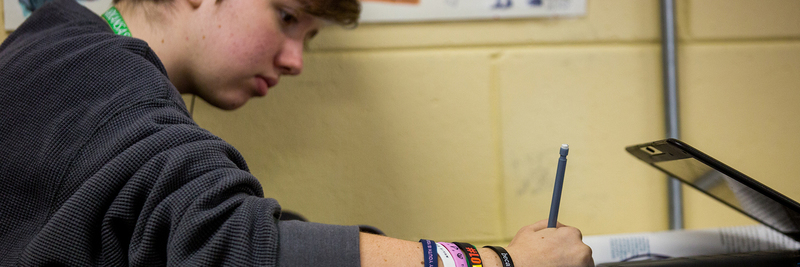EDIT MAINHigh school mathematics begins with a course in algebra where the abstractness of variable notions is introduced and exhausted. The study of one and two variable equations is examined analytically, graphically, and tabularly.

Geometry is the second course, usually taken the sophomore year. Geometry introduces the algebra of shapes in two and three dimensions.

Algebra II is the third course, usually taken the junior year. It introduces complex functions and their properties as well as the algebra of three-space.

After Algebra II, students get to choose a route of study that includes Algebra III, Transitional Math, or Precalculus. Algebra III is commonly referred to as College Algebra. Precalculus further develops pertinent algebra skills as well as the comprehensive study of introductory trigonometry.

We also offer AP Calculus AB and AP Statistics. AP Statistics can be taken in conjunction with Algebra II or Algebra III. AP Calculus can only be taken after a student successfully takes the prerequisite Precalculus course. AP Calculus is the study of rates of change, motion, and accumulation in real world situations.